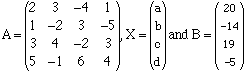Subject: matrices Right now, we are dealing with matrices and we are supposed to solve the following problem on our graphing-calculators: 2a+3b-4c+d=20  a-2b+3c-5d=-14 3a+4b-2c+3d=19 5a-b+6c+4d=-5 I am a student. Hi, I think that what you are expected to do is to write the system of equations as a matrix equation. LetThen your system of equations can be written A X = B To solve this matrix equation you can multiply both sides, on the left, by A-1. That is A-1 A X = A-1 B But A-1 A is the 4-by-4 identity matrix I and I X = X so X = A-1B Using your calculator, construct A and B, compute A-1 and multiply to get A-1B. Cheers, Penny Go to Math Central